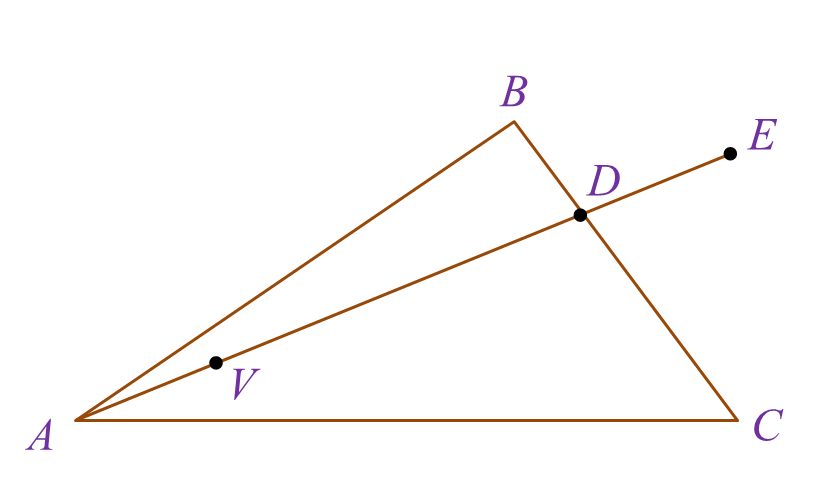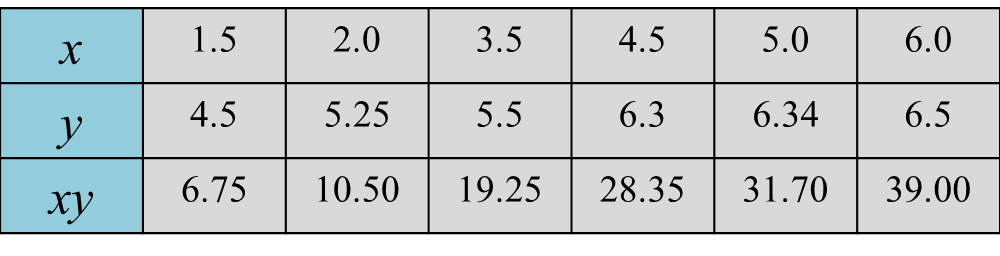\

# SPM Additional Mathematics 2017, Paper 2 (Question 8 & 9)

Question 8 (10 marks):
Diagram 5 shows a triangle ABC. The straight line AE intersects with the straight line BC at point D. Point V lies on the straight line AE.Solution:
(a)(i)

(a)(ii)

(b)

(c)

Question 9 (10 marks):
Use a graph to answer this question.
Table 1 shows the values of two variables, x and y, obtained from an experiment.
The variables x and y are related by the equation $y-\sqrt{h}=\frac{hk}{x}$ , where h and k are constants.(a) Plot xy against x, using a scale of 2 cm to 1 unit on the x-axis and 2 cm to 5 units on the xy-axis.
Hence, draw the line of best fit.
(b) Using the graph in 9(a), find
(i) the value of h and of k,
(ii) the correct value of y if one of the values of y has been wrongly recorded during the experiment.

Solution:
(a)(b)

(b)(i)
$\begin{array}{l}m=\frac{36.5}{5.1}\\ \sqrt{h}=\frac{36.5}{5.1}\\ \sqrt{h}=7.157\\ h=51.22\\ \\ C=-4\\ hk=-4\\ k=\frac{-4}{h}\\ k=-\frac{4}{51.22}\\ k=-0.0781\end{array}$

(b)(ii)